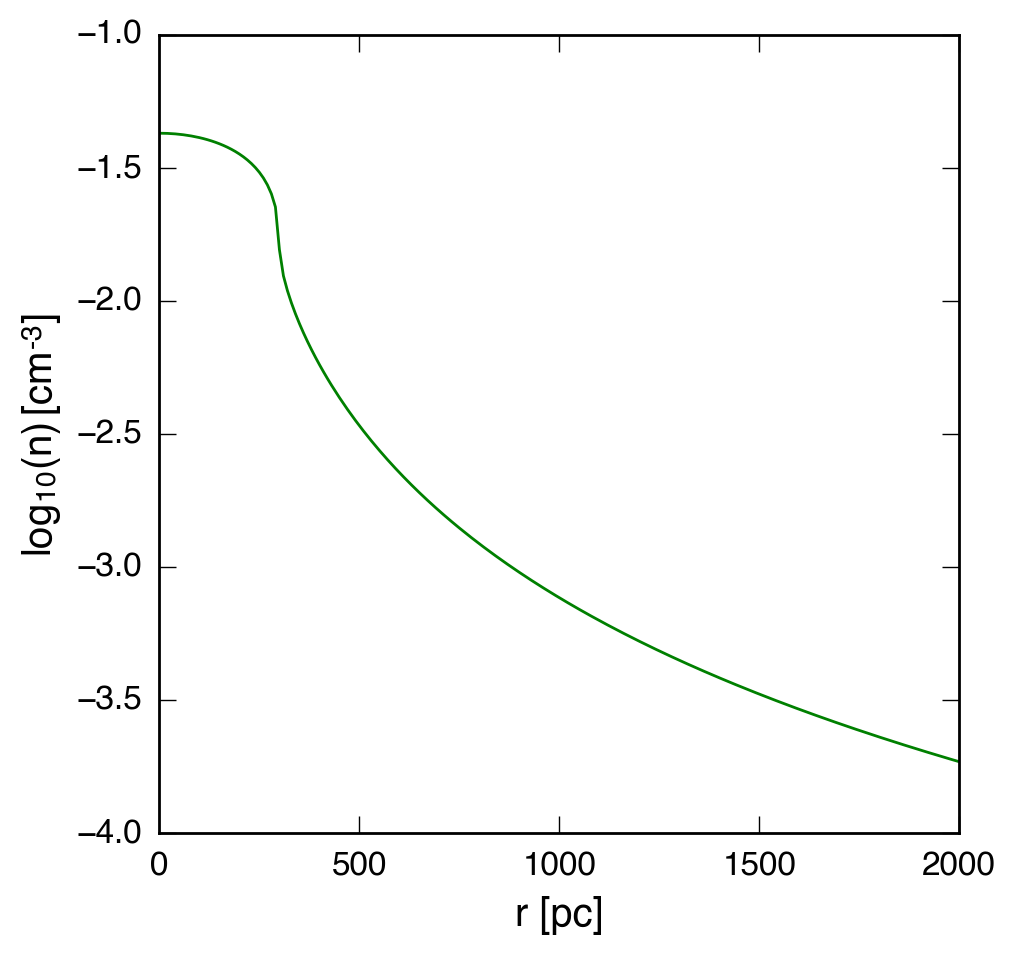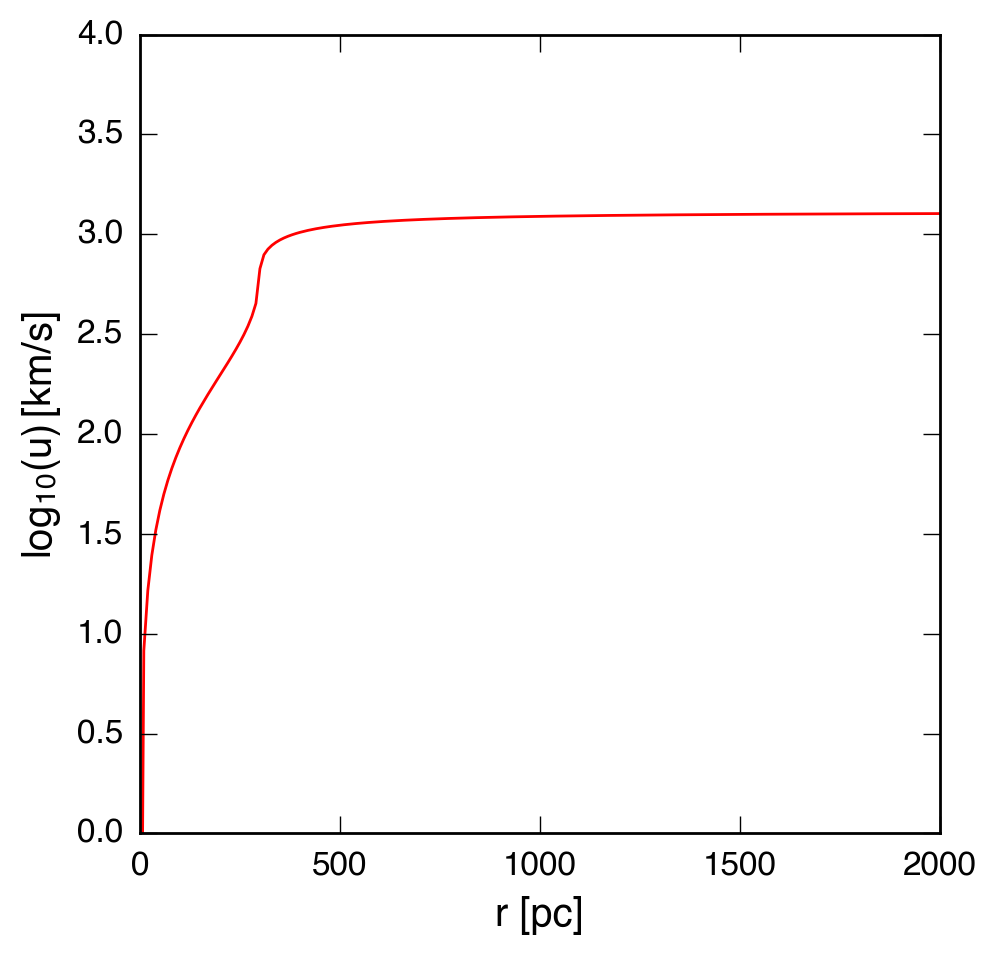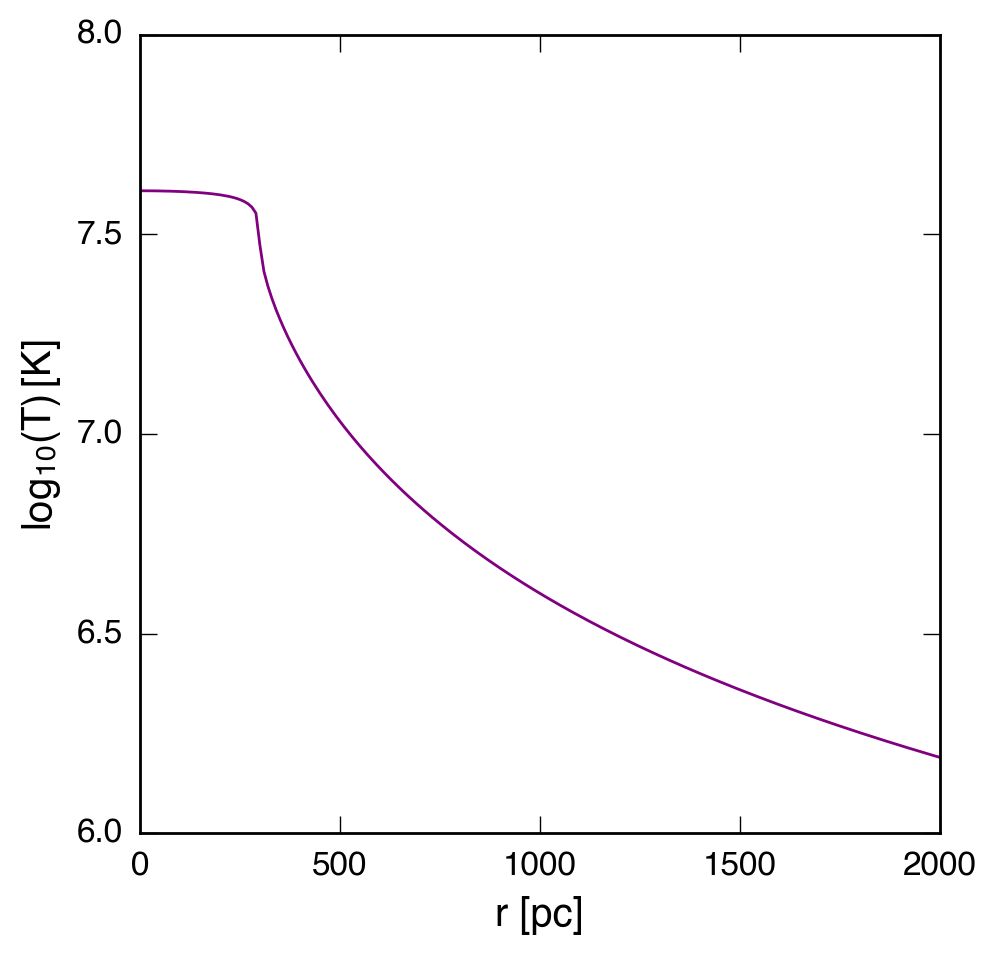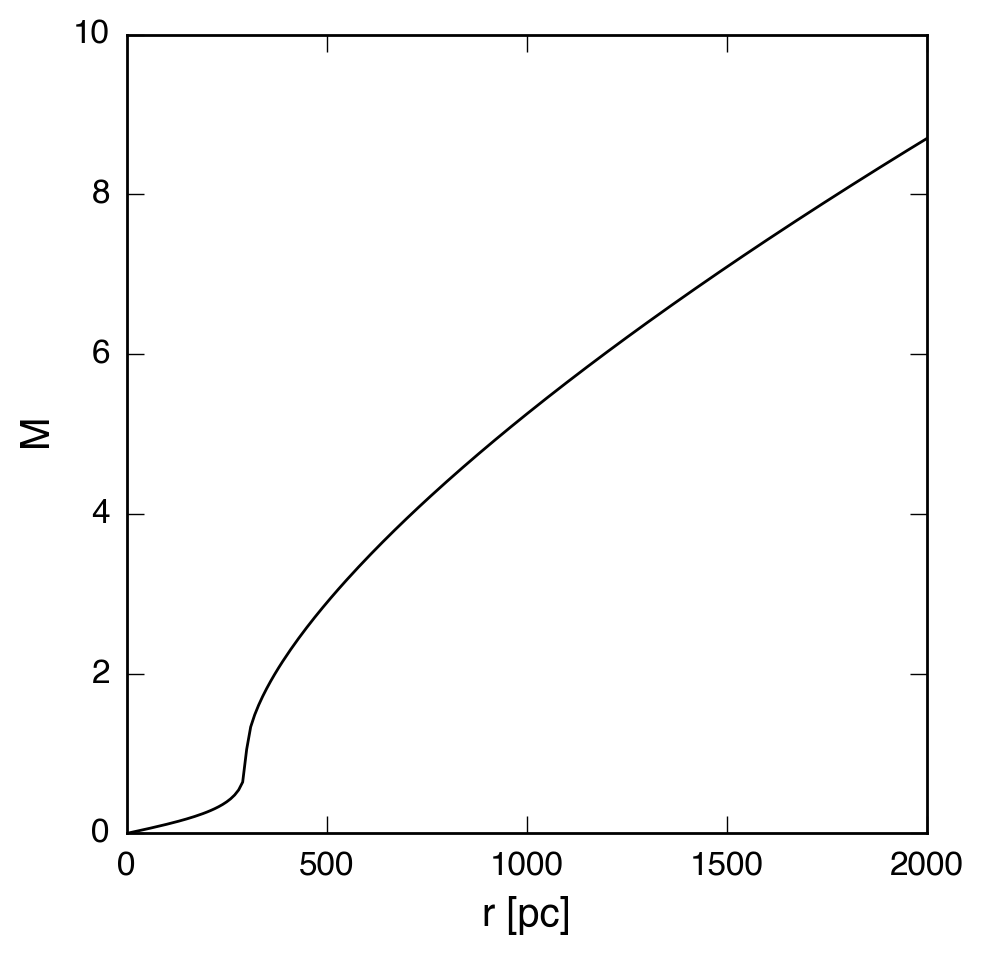Chevalier & Clegg Wind Model (Part 2)

January 20, 2016

For M82, measurements have been made of the parameters that determine the physical properties of the Chevalier and Clegg model (CC85). In Strickland & Heckman (2009), fitting measurements of the hot x-ray gas within the starburst region to 1D models resulted in the following parameters:

• $\dot{M}_{hot} \approx 0.3 \times \beta_{hot} \, \mathrm{M}_{\odot} \, \mathrm{yr}^{-1}$, with $\beta_{hot}$ in the range 1.0 - 2.8
• $\dot{E}_{hot} \approx 6.5 \times 10^{41} \, \mathrm{erg} \, \mathrm{s}^{-1}$, with $\epsilon$ in the range 0.3-1.0
• $R_{*} \approx 300$ pc

Physical parameters of interest for the CC85 model with these parameters are shown in the plots below.